### Numbers

JSONiq follows the W3C standard for constructing numbers. The following explanations, provided as an informal summary for convenience, are non-normative.

Figure 11. Literal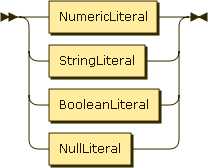Figure 12. NumericLiteral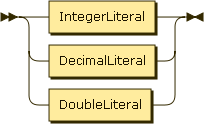Figure 13. IntegerLiteral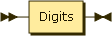Figure 14. DecimalLiteral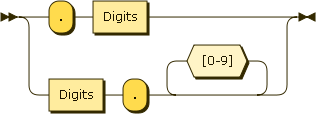Figure 15. DoubleLiteral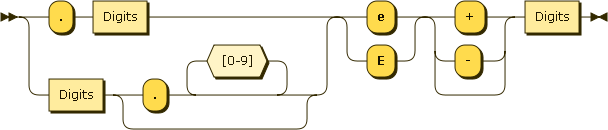The syntax for creating numbers is identical to that of JSON (it is actually a more flexible superset, for example leading 0s are allowed, and a decimal literal can begin with a dot). Note that JSONiq distinguishes between integers (no dot, no scientific notation), decimals (dot but no scientific notation) and doubles (scientific notation). As expected, an integer literal creates an atomic of type integer, and so on.

Example 16. Integer literals

```42
```

Result: 42

Example 17. Decimal literals

```3.14
```

Result: 3.14

Example 18. Double literals

```+6.022E23
```

Result: 6.022E23